## 极坐标下的二重积分，化为二次积分后，每次积分的几何意义是什么？

1 厘清问题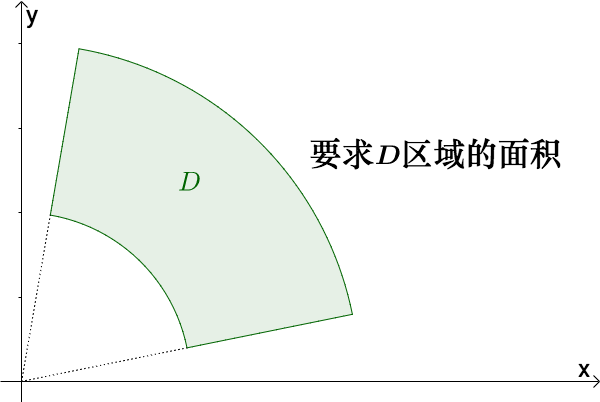$$\displaystyle \mathop {\iint }_{D}dxdy=\mathop {\iint }_{D'}\rho d\rho d\theta$$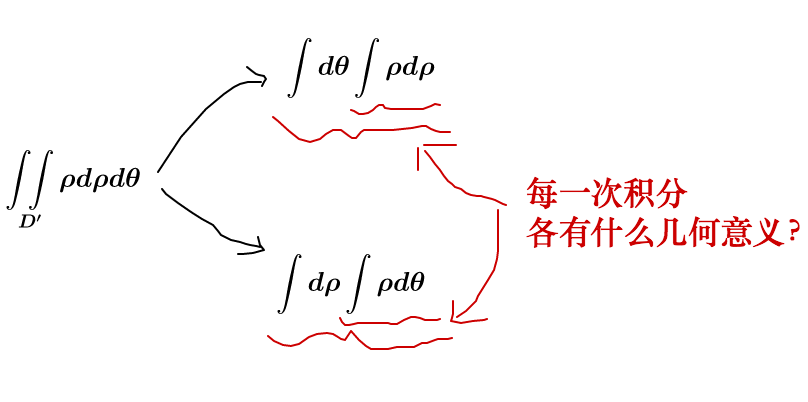2 先给一个直观的回答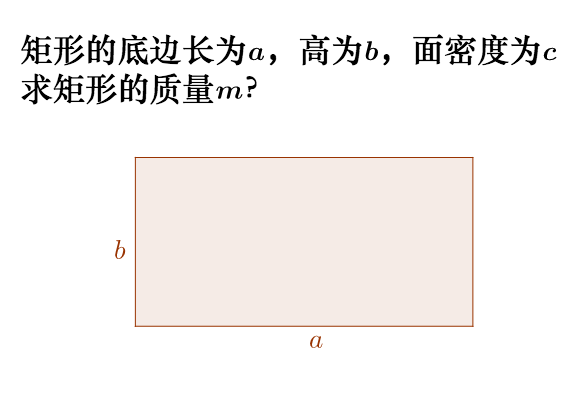$$m=(a\times b)\times c$$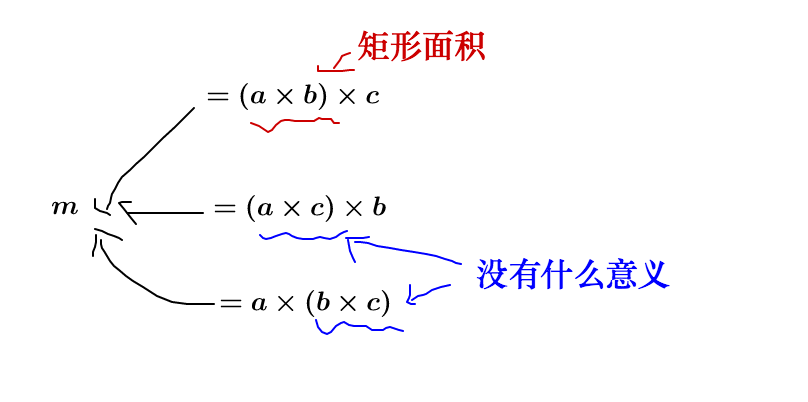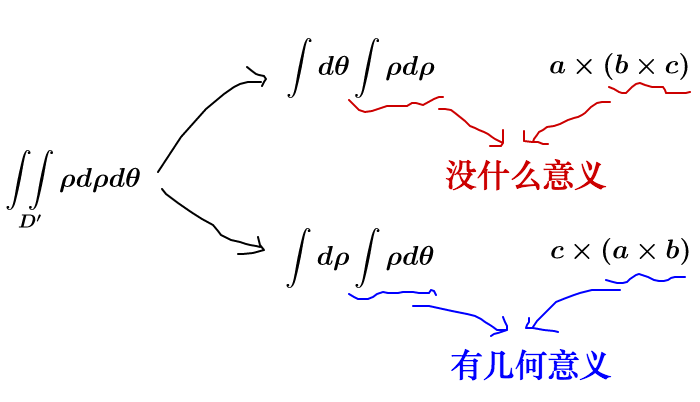3 更深入一点的回答

3.1 不同积分区域下算出来的面积不同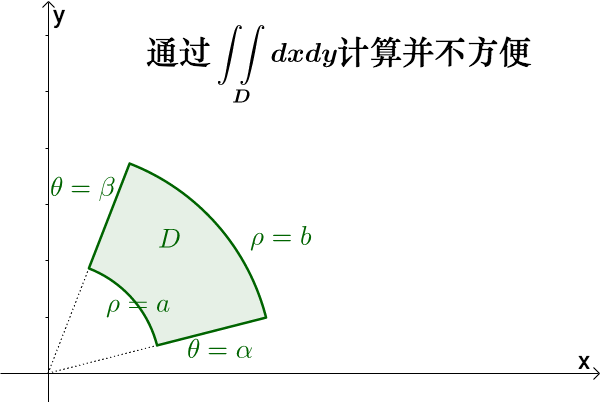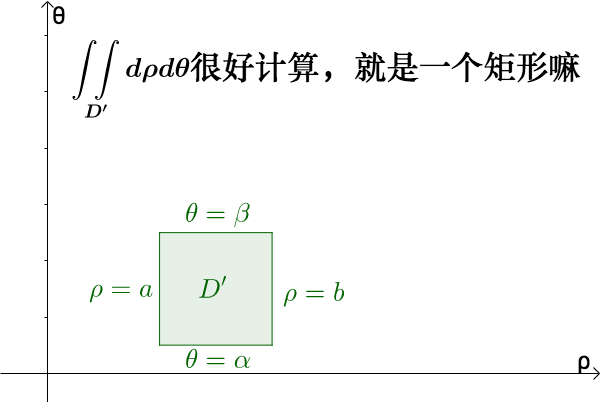$$\displaystyle \mathop {\iint }_{D}dxdy\ne \mathop {\iint }_{D'}d\rho d\theta$$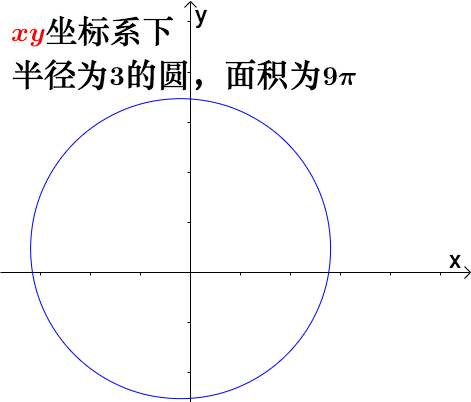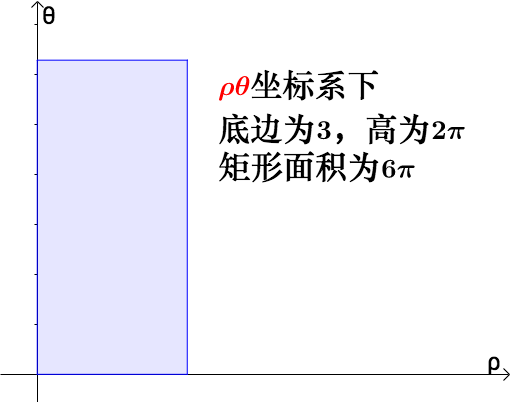3.2 “单位换算”求解的思路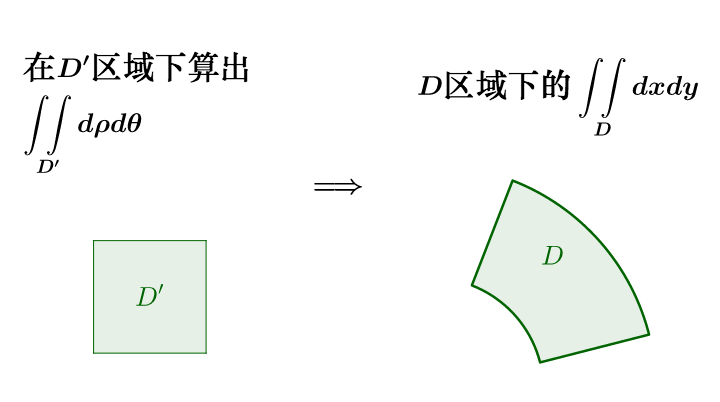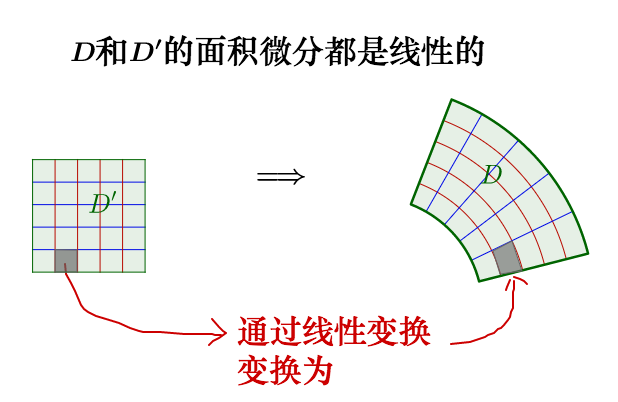3.3 具体的计算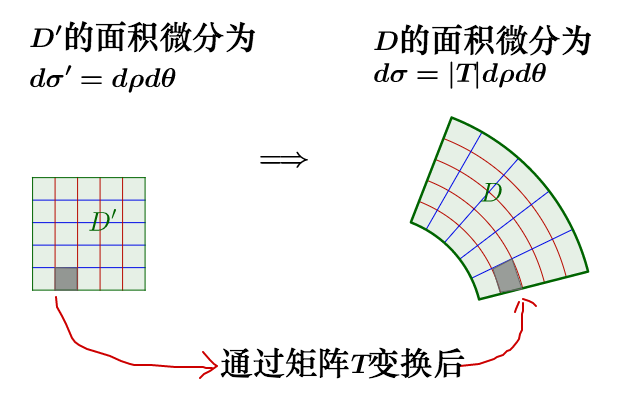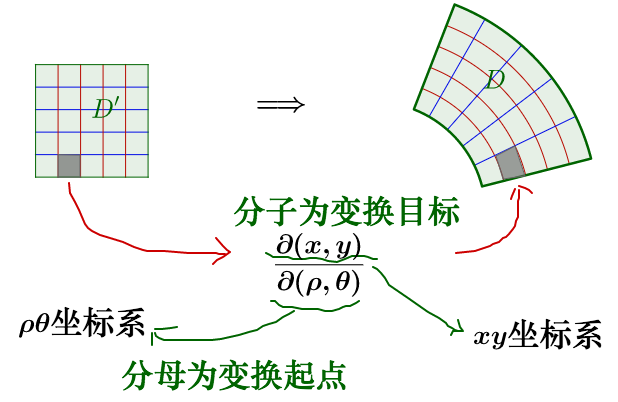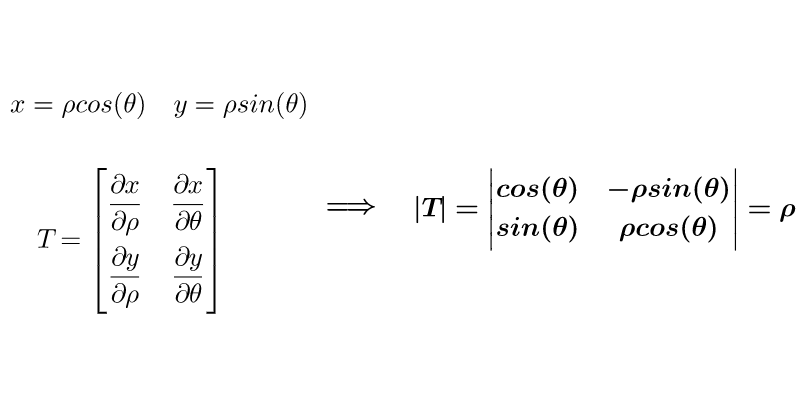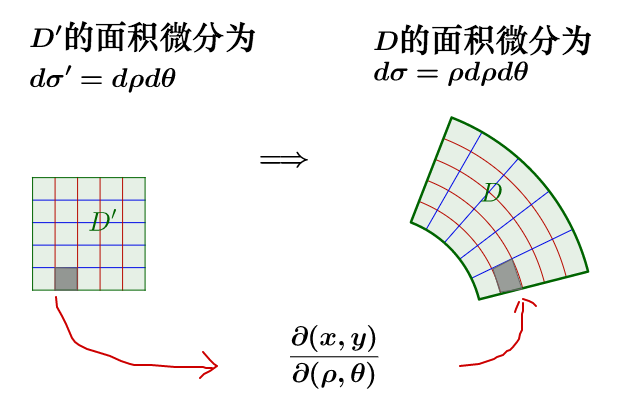4 关于换元进一步的例子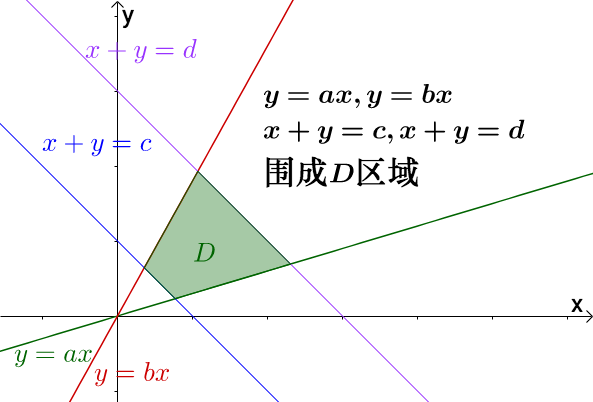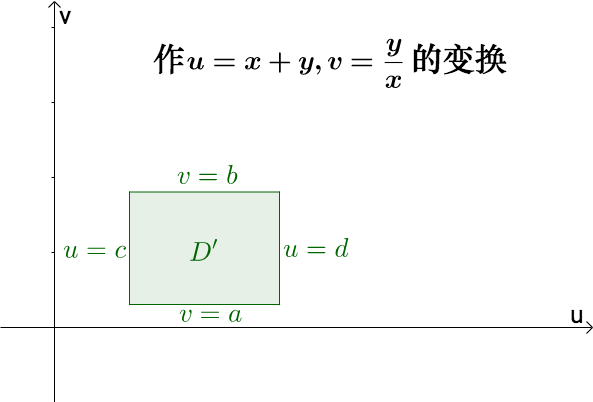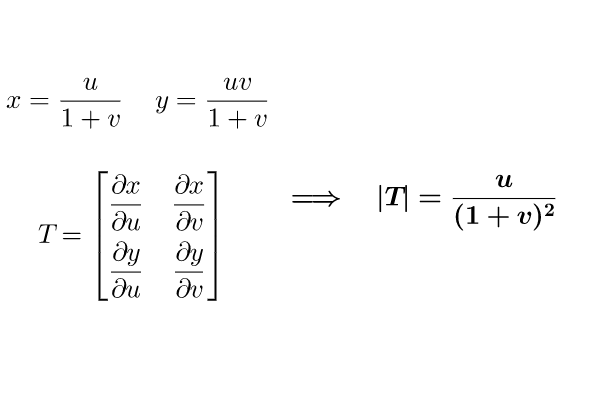$$\displaystyle \mathop {\iint }_{D}dxdy=\mathop {\iint }_{D'}\frac{u}{(1+v)^2}dudv$$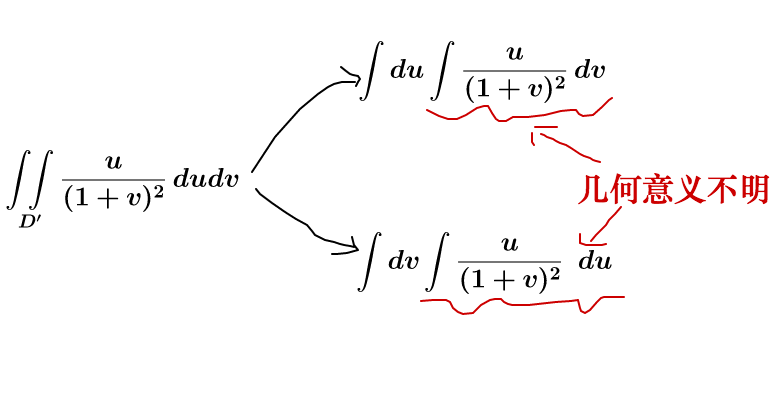5 最后

Created with GeoGebra